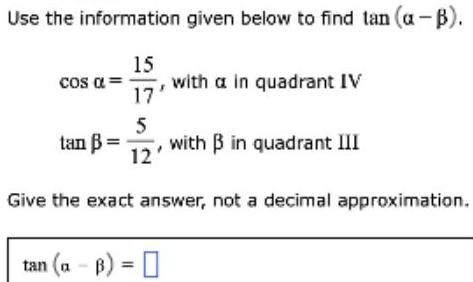Question:

# Use the information given below to find tan (a-B). Cos α =

Last updated: 7/4/2022Use the information given below to find tan (a-B). Cos α = 15/17 with a in quadrant IV tan B=5/12 with B in quadrant III Give the exact answer, not a decimal approximation. tan (a-B) =